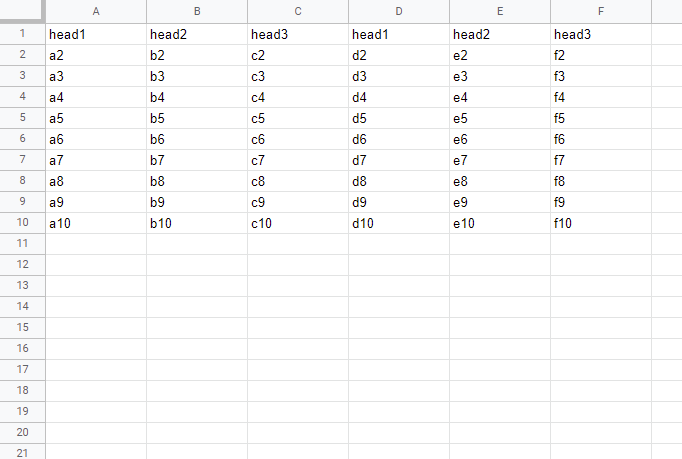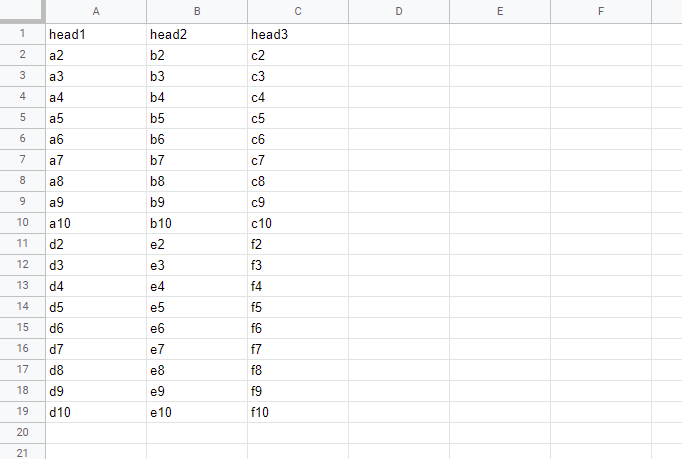Gists

This is a sample Google Apps Script for processing the values in Google Spreadsheet. In this sample situation, each column are merged using the same header title.

In this sample script, the sample input and output situations are as follows.

## Sample situation

### Input: “Sheet1”### Output: “Sheet2”## Sample script

function myFunction() {
const srcSheetName = "Sheet1"; // This sheet is "Input" situation.
const dstSheetName = "Sheet2"; // This sheet is "Output" situation.

const transpose = (ar) => ar.map((_, c) => ar.map((r) => r[c]));
const [src, dst] = [srcSheetName, dstSheetName].map((s) =>
ss.getSheetByName(s)
);
const values = src.getDataRange().getValues();
const temp = [
...transpose(values)
.reduce(
(m, [a, ...b]) => m.set(a, m.has(a) ? [...m.get(a), ...b] : [a, ...b]),
new Map()
)
.values(),
];
const res = transpose(temp);
dst.getRange(1, 1, res.length, res.length).setValues(res);
}

• In this sample script, the following flow is used.
1. Retrieve values from “Sheet1”.
2. Tanspose the retrieved values.
3. Create an array using Map object.
4. Tanspose the created array.
5. Put the array to “Sheet2”.